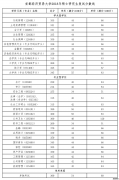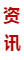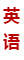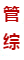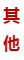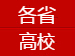# 2019联考数学复习之理出思维的框架

搭出思维的框架就像写文章一样，具体内容还没想全，但头脑中已经有提纲。比如已知等差数列的第二项和第七项，求数列第101项到第200项的和。在具体求之前，头脑中就要先有解题的框架：设数列首项a1和公差d为未知数―》列出两个方程―》解出a1,d―》由数列通项公式计算前N项和公式―》计算S100和S200―》S200-S100得出答案。这样思路清晰，能提高解题速度。

此外，还可以学习一些通用解法。通用解法可以解决相同类型的所有题目，无须再费时间思考。比如线代中的线性方程解法、高数中复合函数的二阶导数、隐函数的偏导数、概率中的数学期望和方差等，都是通用解法，答题的速度和准确性依赖于自己的计算能力，虽然计算复杂，但不用花时间思考。

例如：已知数列通项公式A(N)，求数列的前N项和S(N)。

这个问题等价于求S(N)的通项公式，而S(N)=S(N-1)+A(N)，这就成为递推数列的问题。

解法是寻找一个数列B(N)，

使S(N)+B(N)=S(N-1)+B(N-1)

从而S(N)=A(1)+B(1)-B(N)

猜想B(N)的方法：把A(N)当作函数求积分，对得出的函数形式设待定系数，利用B(N)-B(N-1)=-A(N)求出待定系数。

例题：求S(N)=2+2*2^2+3*2^3+...+N*2^N

解：S(N)=S(N-1)+N*2^N

N*2^N积分得(N*LN2-1)*2^N/(LN2)^2

因此设B(N)=(PN+Q)*2^N

则 (PN+Q)*2^N-[P(N-1)+Q)*2^(N-1)=-N*2^N

(P*N+P+Q)/2*2^N=-N*2^N

因为上式是恒等式，所以P=-2，Q=2

B(N)=(-2N+2)*2^N

A(1)=2，B(1)=0

因此：S(N)=A(1)+B(1)-B(N)

=(2N-2)*2^N+2

对于求集合元素个数的问题，也有通用解法。比如三个相交的集合，可以先画出三个相交的圆圈，分别作为集合A、B、C，A在上，B在左下，C在右下。则A、B、C都被分为四部分，一共分为7块。从上开始，沿逆时针方向将周围一圈设为X1、X2.......X6，中间为X7，AUBUC的补集设为X8。那么题目中给出的任何条件都可以化成关于这八个未知数的方程组，然后变成解线性方程组的问题。如果不用这种方法，题目中的A与B的交集并上C、A与B的差交C等变化万千的条件容易把人搅得头晕脑涨。

与通用解法相对应的是特殊解法。特殊解法方法巧妙，计算简便，可以大大提高解题速度。但掌握特殊解法需要靠大量的练习、总结、积累。如求函数f(x)=x^2(1-x)在[0，1]上的大值，可利用几何平均数小于算术平均数的性质，直接得出：f(x)= x^2(1-x)=4*x/2*x/2*(1-x)<=4*[(x/2+x/2+1-x)/3]^3=4/27，等号在x/2=1-x，即x=2/3时成立。从而大值为4/27。无须求导数、驻点再代入原式计算。

免责声明：本文内容均来源于网友提供或各大相关网站。由本站编辑整理，仅供个人研究、交流学习使用，不涉及商业盈利目的。

(责任编辑：MPAcc考研网)

### 推荐文章### 报考指南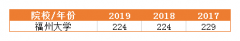### 复试录取